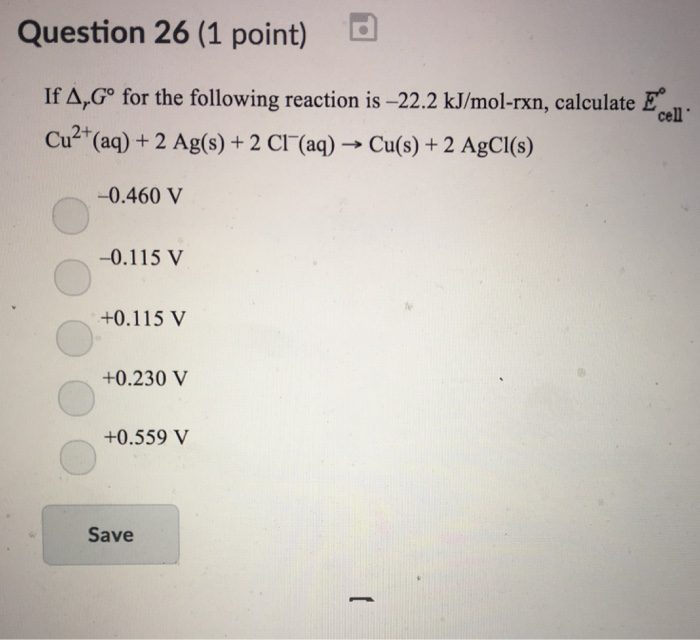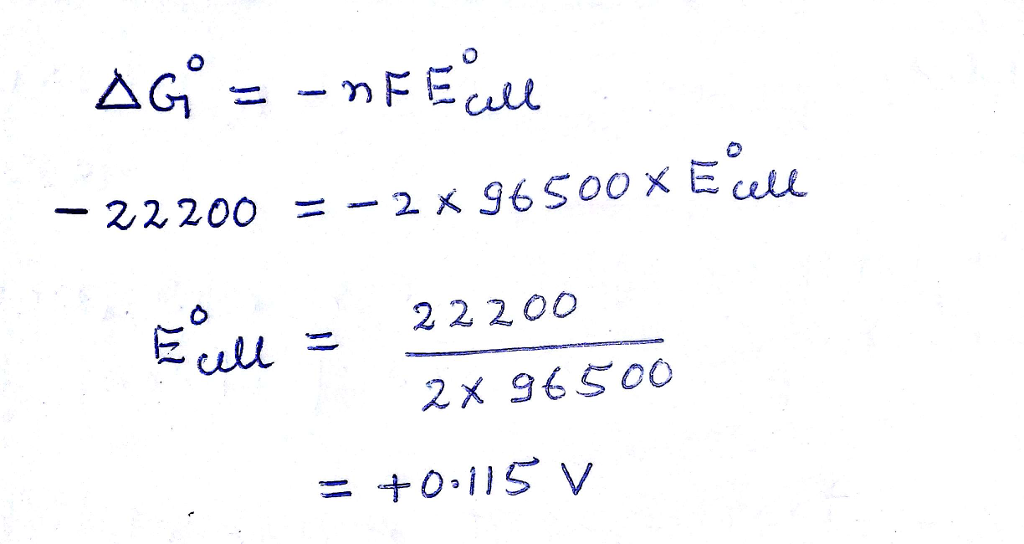# Question & Answer: If Delta_x G degree for the following reaction is -22.2 kJ/mol-rxn, calculate E_cell degree……If Delta_x G degree for the following reaction is -22.2 kJ/mol-rxn, calculate E_cell degree. Cu^2+ (aq) + 2 Ag(s) + 2 Cl^- (aq) rightarrow Cu(s) + 2 AgCl(s) -0.460 V -0.115 V +0.115 V +0.230V +0.559 V